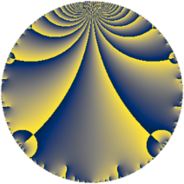# Properties

 Label 735.4.aLevel $735$ Weight $4$ Character orbit 735.a Rep. character $\chi_{735}(1,\cdot)$ Character field $\Q$ Dimension $82$ Newform subspaces $29$ Sturm bound $448$ Trace bound $11$

# Related objects

## Defining parameters

 Level: $$N$$ $$=$$ $$735 = 3 \cdot 5 \cdot 7^{2}$$ Weight: $$k$$ $$=$$ $$4$$ Character orbit: $$[\chi]$$ $$=$$ 735.a (trivial) Character field: $$\Q$$ Newform subspaces: $$29$$ Sturm bound: $$448$$ Trace bound: $$11$$ Distinguishing $$T_p$$: $$2$$, $$11$$, $$13$$

## Dimensions

The following table gives the dimensions of various subspaces of $$M_{4}(\Gamma_0(735))$$.

Total New Old
Modular forms 352 82 270
Cusp forms 320 82 238
Eisenstein series 32 0 32

The following table gives the dimensions of the cuspidal new subspaces with specified eigenvalues for the Atkin-Lehner operators and the Fricke involution.

$$3$$$$5$$$$7$$FrickeDim.
$$+$$$$+$$$$+$$$$+$$$$11$$
$$+$$$$+$$$$-$$$$-$$$$10$$
$$+$$$$-$$$$+$$$$-$$$$7$$
$$+$$$$-$$$$-$$$$+$$$$13$$
$$-$$$$+$$$$+$$$$-$$$$9$$
$$-$$$$+$$$$-$$$$+$$$$11$$
$$-$$$$-$$$$+$$$$+$$$$13$$
$$-$$$$-$$$$-$$$$-$$$$8$$
Plus space$$+$$$$48$$
Minus space$$-$$$$34$$

## Trace form

 $$82 q - 4 q^{2} + 354 q^{4} - 6 q^{6} + 36 q^{8} + 738 q^{9} + O(q^{10})$$ $$82 q - 4 q^{2} + 354 q^{4} - 6 q^{6} + 36 q^{8} + 738 q^{9} - 50 q^{10} + 52 q^{11} + 24 q^{12} - 64 q^{13} + 30 q^{15} + 1338 q^{16} + 24 q^{17} - 36 q^{18} + 248 q^{19} + 40 q^{20} + 196 q^{22} + 368 q^{23} - 342 q^{24} + 2050 q^{25} - 84 q^{26} - 312 q^{29} + 60 q^{30} - 200 q^{31} + 1124 q^{32} - 36 q^{33} + 780 q^{34} + 3186 q^{36} + 1152 q^{37} + 1040 q^{38} + 1020 q^{39} - 450 q^{40} - 372 q^{41} + 1512 q^{43} + 1468 q^{44} + 1480 q^{46} - 504 q^{47} + 912 q^{48} - 100 q^{50} - 780 q^{51} + 296 q^{52} - 1184 q^{53} - 54 q^{54} - 740 q^{55} - 528 q^{57} + 244 q^{58} + 180 q^{59} - 90 q^{60} + 988 q^{61} + 384 q^{62} + 7234 q^{64} + 580 q^{65} - 996 q^{66} - 400 q^{67} + 2936 q^{68} + 1152 q^{69} + 152 q^{71} + 324 q^{72} - 2124 q^{73} + 140 q^{74} + 3936 q^{76} + 3984 q^{78} - 4768 q^{79} + 1200 q^{80} + 6642 q^{81} + 1984 q^{82} - 3368 q^{83} + 780 q^{85} + 12208 q^{86} - 1164 q^{87} + 9148 q^{88} + 2036 q^{89} - 450 q^{90} + 8872 q^{92} - 2304 q^{93} + 288 q^{94} - 520 q^{95} - 2262 q^{96} + 3196 q^{97} + 468 q^{99} + O(q^{100})$$

## Decomposition of $$S_{4}^{\mathrm{new}}(\Gamma_0(735))$$ into newform subspaces

Label Dim. $$A$$ Field CM Traces A-L signs $q$-expansion
$$a_2$$ $$a_3$$ $$a_5$$ $$a_7$$ 3 5 7
735.4.a.a $$1$$ $$43.366$$ $$\Q$$ None $$-4$$ $$-3$$ $$-5$$ $$0$$ $$+$$ $$+$$ $$-$$ $$q-4q^{2}-3q^{3}+8q^{4}-5q^{5}+12q^{6}+\cdots$$
735.4.a.b $$1$$ $$43.366$$ $$\Q$$ None $$-4$$ $$3$$ $$5$$ $$0$$ $$-$$ $$-$$ $$-$$ $$q-4q^{2}+3q^{3}+8q^{4}+5q^{5}-12q^{6}+\cdots$$
735.4.a.c $$1$$ $$43.366$$ $$\Q$$ None $$0$$ $$3$$ $$-5$$ $$0$$ $$-$$ $$+$$ $$-$$ $$q+3q^{3}-8q^{4}-5q^{5}+9q^{9}+42q^{11}+\cdots$$
735.4.a.d $$1$$ $$43.366$$ $$\Q$$ None $$1$$ $$-3$$ $$-5$$ $$0$$ $$+$$ $$+$$ $$-$$ $$q+q^{2}-3q^{3}-7q^{4}-5q^{5}-3q^{6}+\cdots$$
735.4.a.e $$1$$ $$43.366$$ $$\Q$$ None $$1$$ $$-3$$ $$-5$$ $$0$$ $$+$$ $$+$$ $$-$$ $$q+q^{2}-3q^{3}-7q^{4}-5q^{5}-3q^{6}+\cdots$$
735.4.a.f $$1$$ $$43.366$$ $$\Q$$ None $$1$$ $$3$$ $$5$$ $$0$$ $$-$$ $$-$$ $$-$$ $$q+q^{2}+3q^{3}-7q^{4}+5q^{5}+3q^{6}+\cdots$$
735.4.a.g $$1$$ $$43.366$$ $$\Q$$ None $$3$$ $$-3$$ $$-5$$ $$0$$ $$+$$ $$+$$ $$+$$ $$q+3q^{2}-3q^{3}+q^{4}-5q^{5}-9q^{6}+\cdots$$
735.4.a.h $$1$$ $$43.366$$ $$\Q$$ None $$3$$ $$3$$ $$5$$ $$0$$ $$-$$ $$-$$ $$-$$ $$q+3q^{2}+3q^{3}+q^{4}+5q^{5}+9q^{6}+\cdots$$
735.4.a.i $$1$$ $$43.366$$ $$\Q$$ None $$3$$ $$3$$ $$5$$ $$0$$ $$-$$ $$-$$ $$-$$ $$q+3q^{2}+3q^{3}+q^{4}+5q^{5}+9q^{6}+\cdots$$
735.4.a.j $$1$$ $$43.366$$ $$\Q$$ None $$5$$ $$3$$ $$-5$$ $$0$$ $$-$$ $$+$$ $$-$$ $$q+5q^{2}+3q^{3}+17q^{4}-5q^{5}+15q^{6}+\cdots$$
735.4.a.k $$2$$ $$43.366$$ $$\Q(\sqrt{17})$$ None $$-7$$ $$6$$ $$10$$ $$0$$ $$-$$ $$-$$ $$-$$ $$q+(-3-\beta )q^{2}+3q^{3}+(5+7\beta )q^{4}+\cdots$$
735.4.a.l $$2$$ $$43.366$$ $$\Q(\sqrt{2})$$ None $$-4$$ $$-6$$ $$-10$$ $$0$$ $$+$$ $$+$$ $$+$$ $$q+(-2+\beta )q^{2}-3q^{3}+(-2-4\beta )q^{4}+\cdots$$
735.4.a.m $$2$$ $$43.366$$ $$\Q(\sqrt{5})$$ None $$-4$$ $$-6$$ $$10$$ $$0$$ $$+$$ $$-$$ $$-$$ $$q+(-2-\beta )q^{2}-3q^{3}+(1+4\beta )q^{4}+\cdots$$
735.4.a.n $$2$$ $$43.366$$ $$\Q(\sqrt{2})$$ None $$-4$$ $$6$$ $$10$$ $$0$$ $$-$$ $$-$$ $$-$$ $$q+(-2+\beta )q^{2}+3q^{3}+(-2-4\beta )q^{4}+\cdots$$
735.4.a.o $$2$$ $$43.366$$ $$\Q(\sqrt{2})$$ None $$-2$$ $$6$$ $$-10$$ $$0$$ $$-$$ $$+$$ $$-$$ $$q+(-1+\beta )q^{2}+3q^{3}+(1-2\beta )q^{4}+\cdots$$
735.4.a.p $$2$$ $$43.366$$ $$\Q(\sqrt{65})$$ None $$1$$ $$-6$$ $$-10$$ $$0$$ $$+$$ $$+$$ $$-$$ $$q+\beta q^{2}-3q^{3}+(8+\beta )q^{4}-5q^{5}-3\beta q^{6}+\cdots$$
735.4.a.q $$2$$ $$43.366$$ $$\Q(\sqrt{41})$$ None $$3$$ $$-6$$ $$10$$ $$0$$ $$+$$ $$-$$ $$-$$ $$q+(1+\beta )q^{2}-3q^{3}+(3+3\beta )q^{4}+5q^{5}+\cdots$$
735.4.a.r $$3$$ $$43.366$$ 3.3.4892.1 None $$3$$ $$-9$$ $$15$$ $$0$$ $$+$$ $$-$$ $$+$$ $$q+(1-\beta _{1})q^{2}-3q^{3}+(-\beta _{1}+\beta _{2})q^{4}+\cdots$$
735.4.a.s $$3$$ $$43.366$$ 3.3.4892.1 None $$3$$ $$9$$ $$-15$$ $$0$$ $$-$$ $$+$$ $$-$$ $$q+(1-\beta _{1})q^{2}+3q^{3}+(-\beta _{1}+\beta _{2})q^{4}+\cdots$$
735.4.a.t $$4$$ $$43.366$$ 4.4.51264.1 None $$-6$$ $$-12$$ $$20$$ $$0$$ $$+$$ $$-$$ $$+$$ $$q+(-2+\beta _{1}+\beta _{2})q^{2}-3q^{3}+(6-\beta _{1}+\cdots)q^{4}+\cdots$$
735.4.a.u $$4$$ $$43.366$$ 4.4.51264.1 None $$-6$$ $$12$$ $$-20$$ $$0$$ $$-$$ $$+$$ $$+$$ $$q+(-2+\beta _{1}+\beta _{2})q^{2}+3q^{3}+(6-\beta _{1}+\cdots)q^{4}+\cdots$$
735.4.a.v $$4$$ $$43.366$$ $$\mathbb{Q}[x]/(x^{4} - \cdots)$$ None $$1$$ $$-12$$ $$20$$ $$0$$ $$+$$ $$-$$ $$-$$ $$q+\beta _{1}q^{2}-3q^{3}+(10+\beta _{2})q^{4}+5q^{5}+\cdots$$
735.4.a.w $$4$$ $$43.366$$ $$\mathbb{Q}[x]/(x^{4} - \cdots)$$ None $$1$$ $$12$$ $$-20$$ $$0$$ $$-$$ $$+$$ $$-$$ $$q+\beta _{1}q^{2}+3q^{3}+(10+\beta _{2})q^{4}-5q^{5}+\cdots$$
735.4.a.x $$5$$ $$43.366$$ $$\mathbb{Q}[x]/(x^{5} - \cdots)$$ None $$-1$$ $$-15$$ $$25$$ $$0$$ $$+$$ $$-$$ $$-$$ $$q-\beta _{1}q^{2}-3q^{3}+(4+\beta _{1}+\beta _{2})q^{4}+\cdots$$
735.4.a.y $$5$$ $$43.366$$ $$\mathbb{Q}[x]/(x^{5} - \cdots)$$ None $$-1$$ $$15$$ $$-25$$ $$0$$ $$-$$ $$+$$ $$+$$ $$q-\beta _{1}q^{2}+3q^{3}+(4+\beta _{1}+\beta _{2})q^{4}+\cdots$$
735.4.a.z $$5$$ $$43.366$$ $$\mathbb{Q}[x]/(x^{5} - \cdots)$$ None $$3$$ $$-15$$ $$-25$$ $$0$$ $$+$$ $$+$$ $$-$$ $$q+(1-\beta _{1})q^{2}-3q^{3}+(5+\beta _{2})q^{4}-5q^{5}+\cdots$$
735.4.a.ba $$5$$ $$43.366$$ $$\mathbb{Q}[x]/(x^{5} - \cdots)$$ None $$3$$ $$15$$ $$25$$ $$0$$ $$-$$ $$-$$ $$+$$ $$q+(1-\beta _{1})q^{2}+3q^{3}+(5+\beta _{2})q^{4}+5q^{5}+\cdots$$
735.4.a.bb $$8$$ $$43.366$$ $$\mathbb{Q}[x]/(x^{8} - \cdots)$$ None $$2$$ $$-24$$ $$-40$$ $$0$$ $$+$$ $$+$$ $$+$$ $$q+\beta _{1}q^{2}-3q^{3}+(6+\beta _{1}+\beta _{2})q^{4}+\cdots$$
735.4.a.bc $$8$$ $$43.366$$ $$\mathbb{Q}[x]/(x^{8} - \cdots)$$ None $$2$$ $$24$$ $$40$$ $$0$$ $$-$$ $$-$$ $$+$$ $$q+\beta _{1}q^{2}+3q^{3}+(6+\beta _{1}+\beta _{2})q^{4}+\cdots$$

## Decomposition of $$S_{4}^{\mathrm{old}}(\Gamma_0(735))$$ into lower level spaces

$$S_{4}^{\mathrm{old}}(\Gamma_0(735)) \cong$$ $$S_{4}^{\mathrm{new}}(\Gamma_0(5))$$$$^{\oplus 6}$$$$\oplus$$$$S_{4}^{\mathrm{new}}(\Gamma_0(7))$$$$^{\oplus 8}$$$$\oplus$$$$S_{4}^{\mathrm{new}}(\Gamma_0(15))$$$$^{\oplus 3}$$$$\oplus$$$$S_{4}^{\mathrm{new}}(\Gamma_0(21))$$$$^{\oplus 4}$$$$\oplus$$$$S_{4}^{\mathrm{new}}(\Gamma_0(35))$$$$^{\oplus 4}$$$$\oplus$$$$S_{4}^{\mathrm{new}}(\Gamma_0(49))$$$$^{\oplus 4}$$$$\oplus$$$$S_{4}^{\mathrm{new}}(\Gamma_0(105))$$$$^{\oplus 2}$$$$\oplus$$$$S_{4}^{\mathrm{new}}(\Gamma_0(147))$$$$^{\oplus 2}$$$$\oplus$$$$S_{4}^{\mathrm{new}}(\Gamma_0(245))$$$$^{\oplus 2}$$